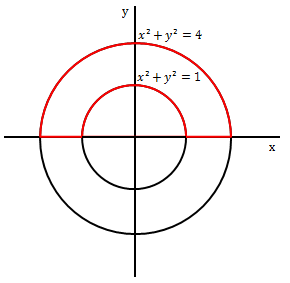# Line integralLet $C$ be the region enclosed by the $x$-axis and the two circles $x^2 + y^2 = 1$ and $x^2+y^2 = 4$ (as shown by the red curves in the figure).

What is the value of $\displaystyle \oint_C \big(y^2 dx + 5xy\, dy\big) ?$

×